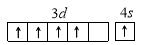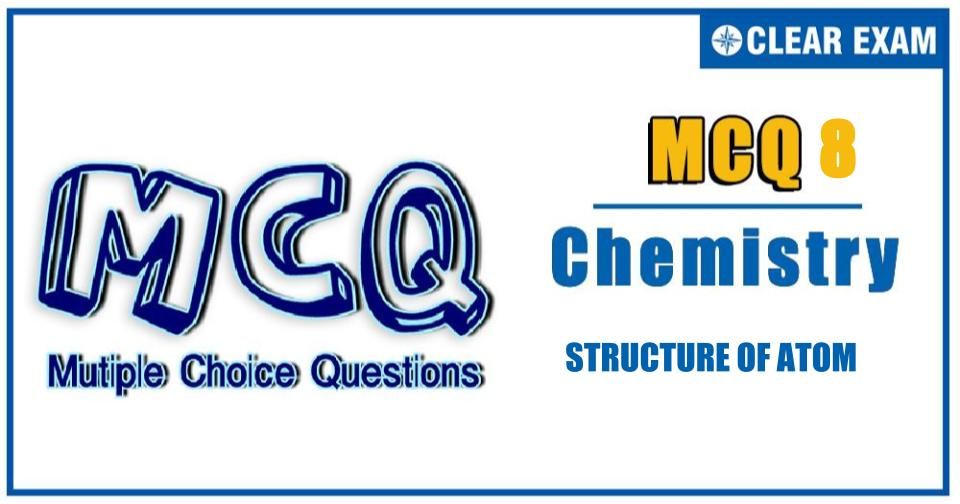[LATEST]\$type=sticky\$show=home\$rm=0\$va=0\$count=4\$va=0

IIT JEE exam which consists of JEE Main and JEE Advanced is one of the most important entrance exams for engineering aspirants. The exam is held for candidates who are aspiring to pursue a career in the field of engineering and technical studies. Chemistry is important because everything you do is chemistry! Even your body is made of chemicals. Chemical reactions occur when you breathe, eat, or just sit there reading. All matter is made of chemicals, so the importance of chemistry is that it's the study of everything..

Q1. Atomic mass of an element is not necessarily a whole number because
•  It contains electrons, protons, and neutrons
•  It exists in allotropic forms
•  It contains isotopes
•  Atoms are no longer indivisible
Solution
Part C

Q2.Which of the following arrangements of electrons is mostly likely to be stable?
••••Solution
Part A

Q3.  A photon of frequency n causes photoelectric emission from a surface with threshold frequency v_0. The de Broglic wavelength λ of the photoelectron emitted is given as
•  Δn=h/2mλ
•  Δn=h/λ
•  [1/v_0 -1/v]=(mc^2)/h
•  λ=√(h/(2m Δn))
SolutionQ4.   The total number of unpaired electrons are
•  1
•  2
•  3
•  4
Solution

(a)
2K, 8L, 9M, and 2N
1s^2 2s^2 2p^6 3s^2 3p^6 3d^1 4s^2
(■(K means n=1,L means n=2 @M means n=3,N means n=4 ))
Structure is 3d^1,4s^2
Atomic number 21
Total number of unpaired d e^-
3d_1=1
Q5.Which of the following is false?
•  Bracket spectral series for which n_1=4 and n_2=5,6,7,… lies in the infrared region of the electromagnetic radiation
•  The orbital 3d_(z^2 ) is symmetrical about z-axis
•  The orbital 3d_xy has no probability of finding electron alongx- and y-axis
•  The orbital 3d_(x^2-y^2 ) has no probability of finding electron along x- and y-axis
SolutionQ6. The energy equivalent to 1 amu is?
•  931.5 MeV
•  93.15 MeV
• 460 MeV
•  554 MeV
Solution
(a) 1 amu=931.5 MeV

Q7.1 g atom of an α-emitting 〖 _Z X〗^A (half life = 10 hr) was placed in sealed containers, 4.52×10^23. Helium atoms will accumulate in the container after
•  4.52 hr
•
•  9.40 hr
•  20.00 hr
Solution
Part D

Q8.The two electrons have the following sets of quantum numbers:
X:3,2,-2,+1/2
Y:3,0,0,+1/2
What is true of the following
•  X and Y have same energy
•  X and Y have unequal energy
•  X and Y represent same electron
•  None of the statement is correct
Solution
(b)
In X; l=d
In Y, l=s
Energy of d>s

Q9.For a given principal level n=4, the energy of its subshells is of the order
•  s< d< f< p
•   s< p< d< f
•  d< f< p< s
•  s< p< f< d
Solution
(b)
s< p< d< f

Q10. The unstable nucleus _82^212 Pbdecays with β - particle emission, having a half-life of 10 h. From this it follows that the
•  I. mass number of the product is 212
•  II. atomic number of the product is 81
•  III. fraction of the original isotope remaining after 20 h is 1/4
• IV. Nucleus formed is stable
Solution
Part BWritten by: AUTHORNAME

AUTHORDESCRIPTIONWant to know more

Please fill in the details below:

Latest NEET Articles\$type=three\$c=3\$author=hide\$comment=hide\$rm=hide\$date=hide\$snippet=hide

Name

ltr
item
BEST NEET COACHING CENTER | BEST IIT JEE COACHING INSTITUTE | BEST NEET, IIT JEE COACHING INSTITUTE: STRUCTURE OF ATOM Quiz-8
STRUCTURE OF ATOM Quiz-8
https://1.bp.blogspot.com/-d3epzeFCg4k/YOgNLleEerI/AAAAAAAA38s/KKcHhS4u2TkFUBtJClsO4u5YgMbDNWZcgCLcBGAsYHQ/s960/Quiz%2BImage%2B20%2B%252832%2529.jpg
https://1.bp.blogspot.com/-d3epzeFCg4k/YOgNLleEerI/AAAAAAAA38s/KKcHhS4u2TkFUBtJClsO4u5YgMbDNWZcgCLcBGAsYHQ/s72-c/Quiz%2BImage%2B20%2B%252832%2529.jpg
BEST NEET COACHING CENTER | BEST IIT JEE COACHING INSTITUTE | BEST NEET, IIT JEE COACHING INSTITUTE
https://www.cleariitmedical.com/2021/07/structure-of-atom-quiz-8.html
https://www.cleariitmedical.com/
https://www.cleariitmedical.com/
https://www.cleariitmedical.com/2021/07/structure-of-atom-quiz-8.html
true
7783647550433378923
UTF-8